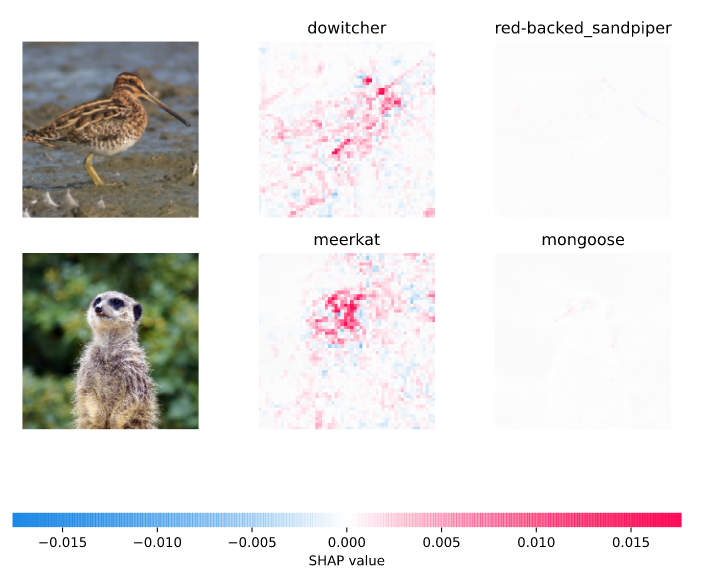# shap

## SHAP Auto-logging¶

If you're already using Lundberg, Lee, et. al.'s SHAP module for Shapley Additive Explanations, then Comet logs the figures out of the box. Simply create SHAP figures as you normally would, and Comet.ML will automatically log them.

For example:

```# import comet_ml before shap
from comet_ml import Experiment

import shap

experiment = Experiment()

# Call any of the figure-producing functions
shap.image_plot(...)
```

That's it for logging the figures!

To see the logged figures in the Comet.ml UI, you can go to the Graphics Tab of the Experiment View.To disable the SHAP auto-logging, set the `comet.auto_log.figures` configuration variable to `False`. For example:

```import os
os.environ["COMET_AUTO_LOG_FIGURES"] = "0"
# SHAP auto-log of figures is now disabled

# import comet_ml before shap
from comet_ml import Experiment

import shap

experiment = Experiment()

# Call any of the figure-producing functions
shap.image_plot(...)
```